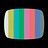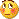# Indicators with alerts/signal - page 596160

Hello

Magic Dots is a repaint indicator ?153863

kayvan:
Hello Magic Dots is a repaint indicator ?

kayvan

It depends how you look at it. What it does is that it shows a signal 1 bar back (on a closed bar) instead of showing signal on an opened bar, and that signal can change. I don't know why the author made it that way, but anyway, I left it work as the original author made it. Once the bar is closed the signal will remain (so after that there will be no change of it - it will not repaint). Anyway, found one issue in the indicator (not cleaning the buffers before the calculation). It is corrected in this version

___________________

PS: regarding the indicator itself - from what I see, you should be carefull in a flat market with it - it will give a lot of signals in a flat market and might be considered as a dangerous indicator in a flat market

Files:9493

handy148:
mrtools - your lovely indi in post #5937 gives an arrow when HA changes colour which is great. Is it possible to get another arrow and alert when price closes >< HA regardless of whether HA has changed colour?

Handy148, Sorry about that, this version looks more like what you are looking for. Because of mt4 buffer restrictions had to make it a separate indicator.

Files:69

Hi team...

Files:9493

endymion:

Hi Endymion,

Have this one its the same except its mtf with alerts also you can choose the ma type on the picture using a 6 period LWMA.

updated version posted here: https://www.mql5.com/en/forum/180648

Files:69

[PHP][/PHP]

mrtools:
Hi Endymion, Have this one its the same except its mtf with alerts also you can choose the ma type on the picture using a 6 period LWMA.

Most excellent!!! Thanks MrTools59

Files:153863

rizkyd:

rizkyd

Decompiled code

Sorry59

rizkyd

Decompiled code

Sorry

int start() {

double ld_16;

double ld_24;

double ld_32;

double ld_40;

double ld_48;

double ld_56;

int li_unused_64;

int li_68;

int li_72;

for (int li_0 = Bars - gi76; li_0 >= 0; li_0--) {

ld_16 = Low;

if (ld_16 == ld_56) ld_16 = 0.0;

else {

ld_56 = ld_16;

if (Low[li_0] - ld_16 > gi80 * Point) ld_16 = 0.0;

else {

for (int li_4 = 1; li_4 <= gi84; li_4++) {

ld_24 = gibuf88[li_0 + li_4];

if (ld_24 != 0.0 && ld_24 > ld_16) gibuf88[li_0 + li_4] = 0.0;

}

}

}

gibuf88[li_0] = ld_16;

ld_16 = High;

if (ld_16 == ld_48) ld_16 = 0.0;

else {

ld_48 = ld_16;

if (ld_16 - High[li_0] > gi80 * Point) ld_16 = 0.0;

else {

for (li_4 = 1; li_4 <= gi84; li_4++) {

ld_24 = gibuf92[li_0 + li_4];

if (ld_24 != 0.0 && ld_24 < ld_16) gibuf92[li_0 + li_4] = 0.0;

}

}

}

gibuf92[li_0] = ld_16;

}

ld_48 = -1;

int li_8 = -1;

ld_56 = -1;

int li_12 = -1;

for (li_0 = Bars - gi76; li_0 >= 0; li_0--) {

ld_32 = gibuf88[li_0];

ld_40 = gibuf92[li_0];

if (ld_32 == 0.0 && ld_40 == 0.0) continue;

if (ld_40 != 0.0) {

if (ld_48 > 0.0) {

if (ld_48 < ld_40) gibuf92[li_8] = 0;

else gibuf92[li_0] = 0;

}

if (ld_48 < ld_40 || ld_48 < 0.0) {

ld_48 = ld_40;

li_8 = li_0;

}

ld_56 = -1;

}

if (ld_32 != 0.0) {

if (ld_56 > 0.0) {

if (ld_56 > ld_32) gibuf88[li_12] = 0;

else gibuf88[li_0] = 0;

}

if (ld_32 < ld_56 || ld_56 < 0.0) {

ld_56 = ld_32;

li_12 = li_0;

}

ld_48 = -1;

}

}

for (li_0 = Bars - 1; li_0 >= 0; li_0--) {

if (li_0 >= Bars - gi76) gibuf88[li_0] = 0.0;

else {

ld_24 = gibuf92[li_0];

if (ld_24 != 0.0) gibuf92[li_0] = ld_24;

}

li_unused_64 = 0;

for (int l_index_76 = 0; gibuf88[l_index_76] == 0.0 && gibuf92[l_index_76] == 0.0; l_index_76++) {

}

li_68 = l_index_76;

for (l_index_76++; gibuf88[l_index_76] == 0.0 && gibuf92[l_index_76] == 0.0; l_index_76++) {

}

li_72 = l_index_76;

if (gi96 != li_68 || gi100 != li_72) {

gi96 = li_68;

gi100 = li_72;

}

if (gi96 != li_68 || gi100 != li_72) {

gi96 = li_68;

gi100 = li_72;

}

}

return (0);

}

thanks....153863

rizkyd:

int start() {

double ld_16;

double ld_24;

double ld_32;

double ld_40;

double ld_48;

double ld_56;

int li_unused_64;

int li_68;

int li_72;

for (int li_0 = Bars - gi76; li_0 >= 0; li_0--) {

ld_16 = Low;

if (ld_16 == ld_56) ld_16 = 0.0;

else {

ld_56 = ld_16;

if (Low[li_0] - ld_16 > gi80 * Point) ld_16 = 0.0;

else {

for (int li_4 = 1; li_4 <= gi84; li_4++) {

ld_24 = gibuf88[li_0 + li_4];

if (ld_24 != 0.0 && ld_24 > ld_16) gibuf88[li_0 + li_4] = 0.0;

}

}

}

gibuf88[li_0] = ld_16;

ld_16 = High;

if (ld_16 == ld_48) ld_16 = 0.0;

else {

ld_48 = ld_16;

if (ld_16 - High[li_0] > gi80 * Point) ld_16 = 0.0;

else {

for (li_4 = 1; li_4 <= gi84; li_4++) {

ld_24 = gibuf92[li_0 + li_4];

if (ld_24 != 0.0 && ld_24 < ld_16) gibuf92[li_0 + li_4] = 0.0;

}

}

}

gibuf92[li_0] = ld_16;

}

ld_48 = -1;

int li_8 = -1;

ld_56 = -1;

int li_12 = -1;

for (li_0 = Bars - gi76; li_0 >= 0; li_0--) {

ld_32 = gibuf88[li_0];

ld_40 = gibuf92[li_0];

if (ld_32 == 0.0 && ld_40 == 0.0) continue;

if (ld_40 != 0.0) {

if (ld_48 > 0.0) {

if (ld_48 < ld_40) gibuf92[li_8] = 0;

else gibuf92[li_0] = 0;

}

if (ld_48 < ld_40 || ld_48 < 0.0) {

ld_48 = ld_40;

li_8 = li_0;

}

ld_56 = -1;

}

if (ld_32 != 0.0) {

if (ld_56 > 0.0) {

if (ld_56 > ld_32) gibuf88[li_12] = 0;

else gibuf88[li_0] = 0;

}

if (ld_32 < ld_56 || ld_56 < 0.0) {

ld_56 = ld_32;

li_12 = li_0;

}

ld_48 = -1;

}

}

for (li_0 = Bars - 1; li_0 >= 0; li_0--) {

if (li_0 >= Bars - gi76) gibuf88[li_0] = 0.0;

else {

ld_24 = gibuf92[li_0];

if (ld_24 != 0.0) gibuf92[li_0] = ld_24;

}

li_unused_64 = 0;

for (int l_index_76 = 0; gibuf88[l_index_76] == 0.0 && gibuf92[l_index_76] == 0.0; l_index_76++) {

}

li_68 = l_index_76;

for (l_index_76++; gibuf88[l_index_76] == 0.0 && gibuf92[l_index_76] == 0.0; l_index_76++) {

}

li_72 = l_index_76;

if (gi96 != li_68 || gi100 != li_72) {

gi96 = li_68;

gi100 = li_72;

}

if (gi96 != li_68 || gi100 != li_72) {

gi96 = li_68;

gi100 = li_72;

}

}

return (0);

}

thanks....

Use any indicator with alerts as a template